At Timestables.com you can easily practice all of your tables. The arithmetic problems are clear and simple so you can immediately get started on practicing your tables. Select one of the multiplication table you wish to practice from the list below and show what you can do on the speed test or printout great worksheets.

## Multiplication games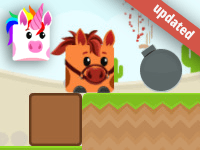Blocky Multiplication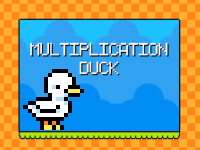Multiplication DuckMy Smart HorseMultiplication JumpMultiplication MageFigo and FriendsRally V10Happy Burger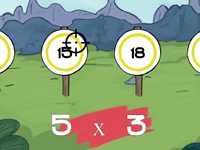Times Tables ShootingTimes Tables RallyNumber DivingFigo's Factory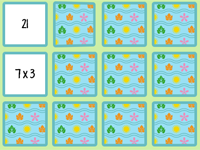Multiplication memory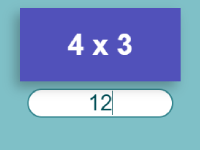Flash cardsMultiplication Cat

Choose the table you want to practice from the following. First you can practice the multiplication facts in sequence and once you have got the hang of that you can practice all the sums in random order for each table. If you have forgotten any answers, just go back to the 'all tables in sequence' page and practice them again thoroughly before trying again.

Once you have got the hang of a number of tables you can select the speed test and choose the tables you want to practice getting quicker at. If you make a mistake, you came see what the right answer is at the end of the test. This will help you learn all of your tables. The speed test is good practice for getting your tables diploma. On the tables diploma the questions are a bit quicker than on the speed test, but if you get all of them right you will get your tables diploma. There are two tables diplomas. The little diploma is made up of 30 questions. Your little diploma shows you can do the 1,2,3,4,5 and 10 times tables. For the big tables diploma you are given 40 questions which include all the tables from 1 to 12.

Learn the multiplication tables in an interactive way with the free math multiplication learning games for 2rd, 3th, 4th and 5th grade. The game element in the times tables games make it even more fun learn.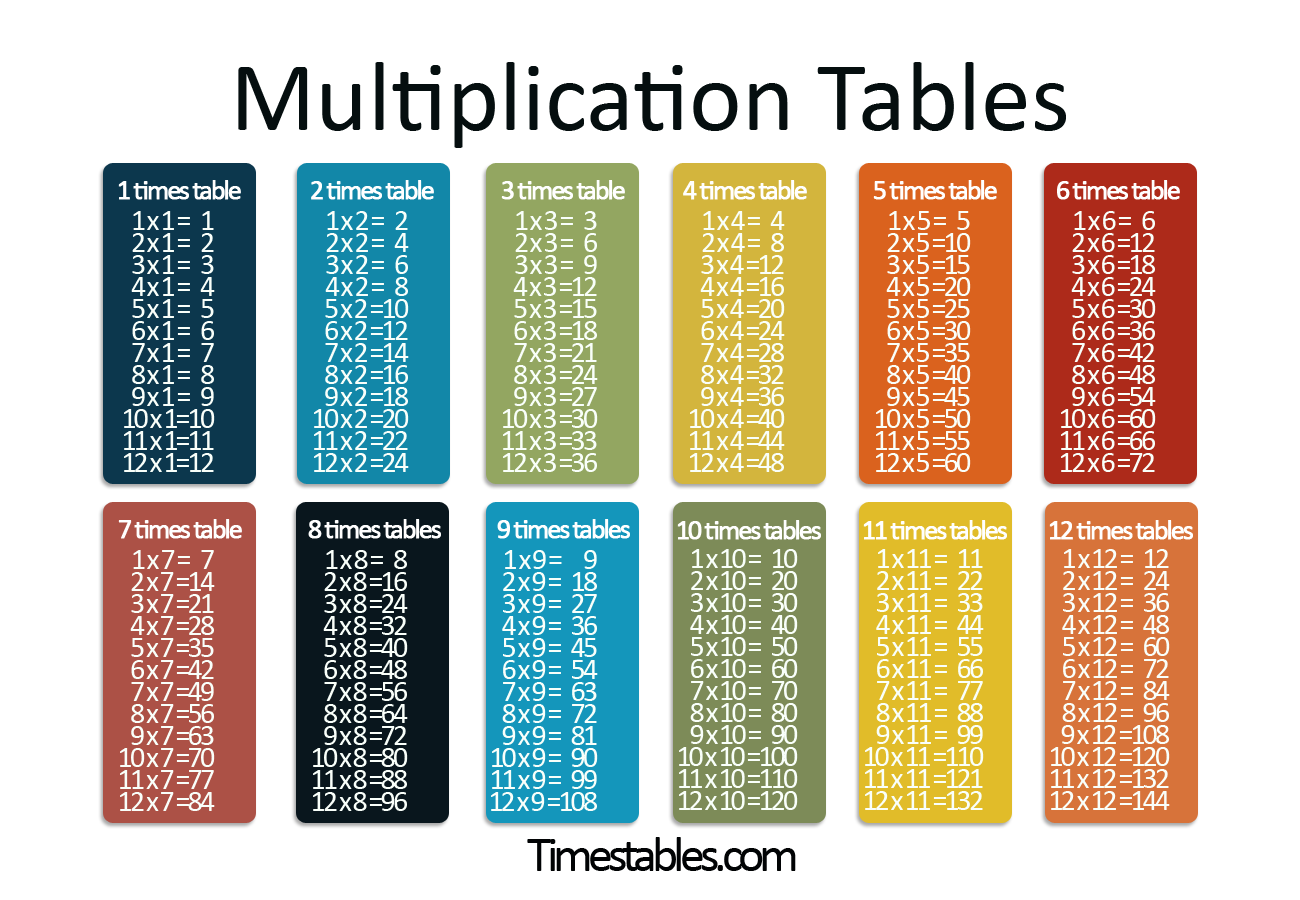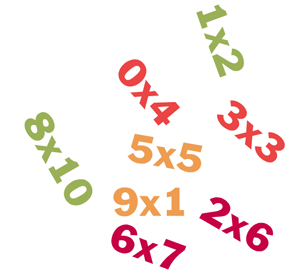Here you can find additional information about practicing multiplication tables at primary school. The 1 times table, 2 times table, 3 times table, 4 times table, 5 times table and 10 times table are the first times tables to be learned. The 6 times table, 7 times table, 8 times table, 9 times table, 11 times table, 12 times table and of course all the tables in random order are the next step.

Not every child finds it easy to learn all the tables, so it is a good idea to keep on practicing them regularly after you learned them. There are a few tricks which make it easier to learn the tables and continue to master them, such as putting the smallest number first, which makes it easier for many children to answer correctly. For example 4 x 9 is easier to work out than 9 x 4. Switching the multiplication sum around makes it easier to answer. What also often helps is to use the tables you know well for answering the sums in the more difficult tables. One example of this is 6 x 7, which is often said to be tricky. If you do 5 x 7 first and then add 1 x 7 it is suddenly easier to answer. You can do this the other way round too. For instance with 4 x 7 you can do 5 x 7 first then subtract 1 x 7.

Why do we put so much effort into learning tables? Well, the multiplication tables keep on coming up in the next primary school years and even when you're at secondary school. You don't see them as tables, but as part of bigger mathematical problems. And it isn't only at school, but in your everyday life it is useful to know your times tables well so you can quickly work things out, for instance when you are buying or selling vegetables on the market. If you have any questions, comments or ideas for Timestables.com, please use our contact form. We'd be glad to hear from you.

## The 5-step plan

Learn the times tables with the 5-step plan. We developed an innovative five step plan to help pupils learn the times tables in an effective and efficient way. This method has been tested at several schools and is recommended by teachers.
The steps are:

• Step 1a: View, read aloud and repeat. To get familiar with the table.
• Step 1b: Fill in your times tables answers in sequence and check if you got them all right.
• Step 2: Drag the correct answers to the questions.
• Step 3: Fill in your answers for the mixed questions and check if you got them all right.
• Step 4: Multiple choice questions will help you to improve by looking at the questions in a different way.
• Step 5: Proof your knowledge and get the diploma.

When you finished the 5 steps you can play the memory game or exercise with the worksheet. Other way to train more are with the tempo test, the 1 minute test or to play the times tables games.

We advise to exercise daily for 15 minutes for maximum result.

How can I learn my times tables fast?
Learn them in chunks. Start with the easy times tables like 10, 2, and 5. Use methods like skip counting, adding and exercising daily for 15 minutes for a maximum long-term result.
What is the hardest times table in the world?
The 7 times table is the hardest to learn because 7 is a prime number, and so the last digit does not repeat itself until 10 times 7. The hardest times table question is 6 times 8.
Which times table is the easiest?
The 10 times table is one of the easiest to learn. For a start, numbers in the 10 times table always end in a 0. And then the 5 and the 2 multiplication tables are also one of the easiest.

## Practice your time tables online

Multiplication tables are important and there are not many places where you can learn them quickly and easily, so we have come up with Timestables.com! Practicing your tables online is really easy at Timestables.com. The multiplication games are clear and simple so you can get started right away. Click on one of the tables to get started right away. Fill in the answers in the lines and when you've finished, click on 'check'. You will see right away which answers are correct and which are incorrect. You can also practice different times tables in one exercise so you can test whether you know them all.

You can also practice the tables interactively with the free multiplication games. On the multiplication games page we now have an answer-dragging game and a table balloons game.

Multiplication tables form the basis for the calculations that you do in the following years, so it’s important that you fully understand them.

Do you want to practice more math? Go to Mathdiploma.com - Here you can practice addition, subtraction, multiplying, dividing and a lot more!

We also have a fraction website! On Fractionsweb.com are lots of exercices about simplifying, adding, subtracting, dividing and multiplying fractions. There are fraction games, worksheets and 5-step plans!